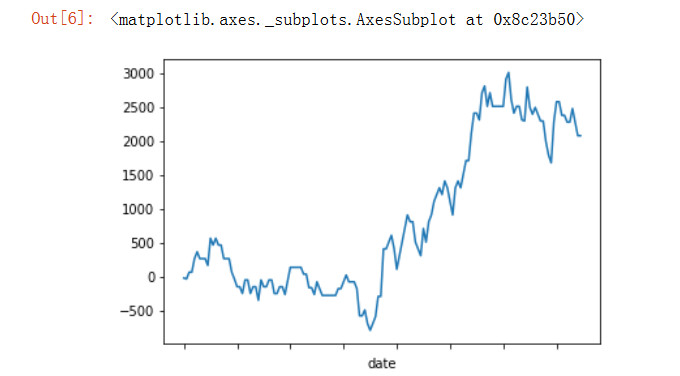Home >  > 从Tushare获取数据展示双均线策略

## 从Tushare获取数据展示双均线策略

0

Tushare是一个财经数据接口包。主要实现对股票等金融数据从数据采集、清洗加工 到 数据存储的过程，能够为金融分析人员提供快速、整洁、和多样的便于分析的数据，为他们在数据获取方面极大地减轻工作量，使他们更加专注于策略和模型的研究与实现上。考虑到Python pandas包在金融量化分析中体现出的优势，Tushare返回的绝大部分的数据格式都是pandas DataFrame类型，非常便于用pandas/NumPy/Matplotlib进行数据分析和可视化。

1、获取数据

```>>> import tushare as ts
>>> df = ts.get_hist_data("510050","2018-01-01")
>>> df = df.sort_values("date")   #原数据2017-01-01排在最后面，所以重新排序
>>> df.to_csv("data.csv")
```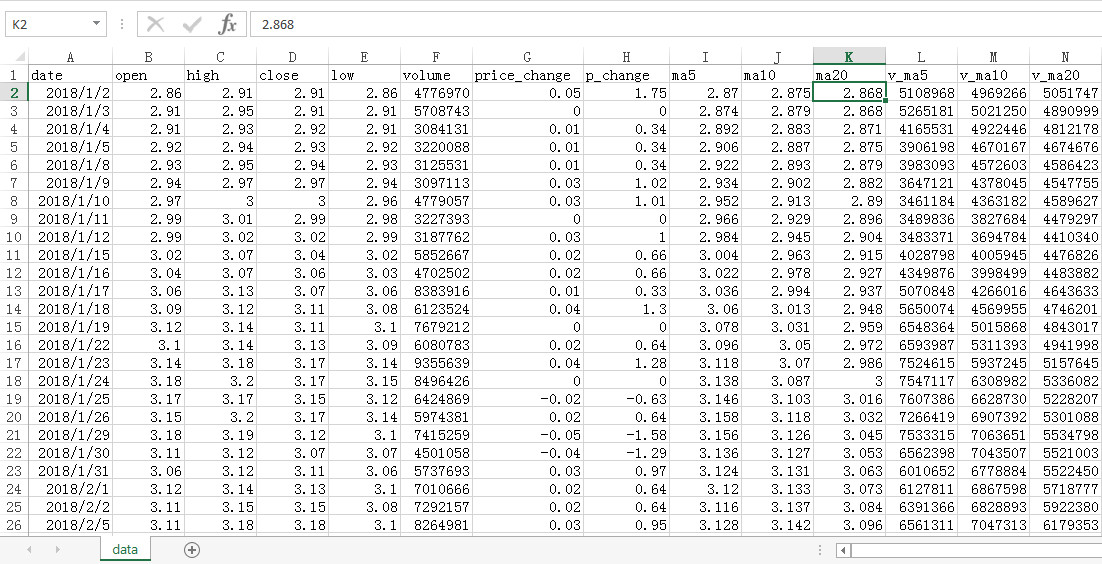2、加工数据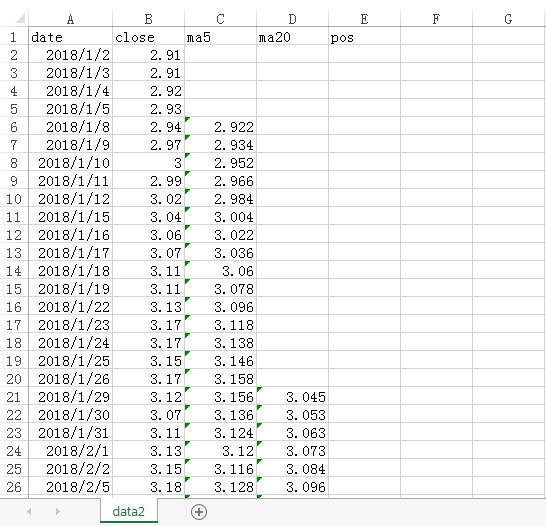3、写策略

```=IF(C21>D21,10000,-10000)     #持仓情况
=ABS(E23-E22)*B23*0.00015     #手续费
```

4、展示数据（2017年01.01-08.31数据）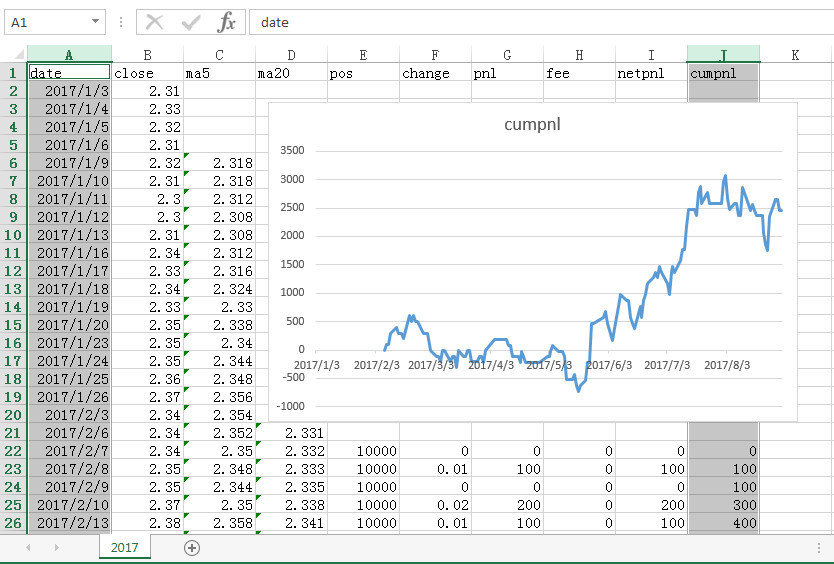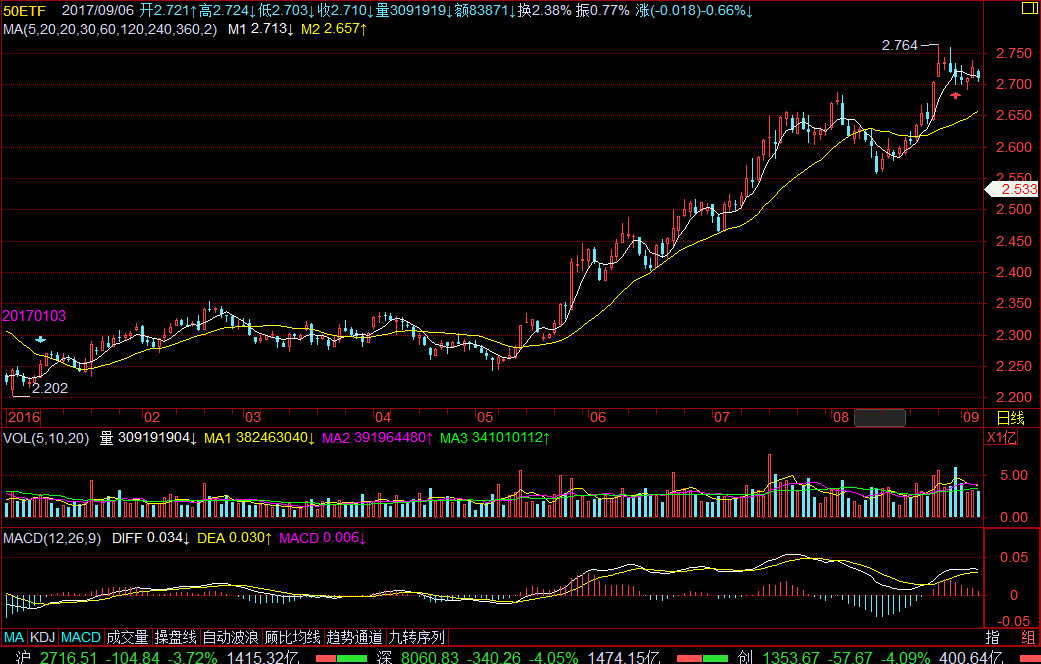5、展示数据（2018年01.01-10.08数据）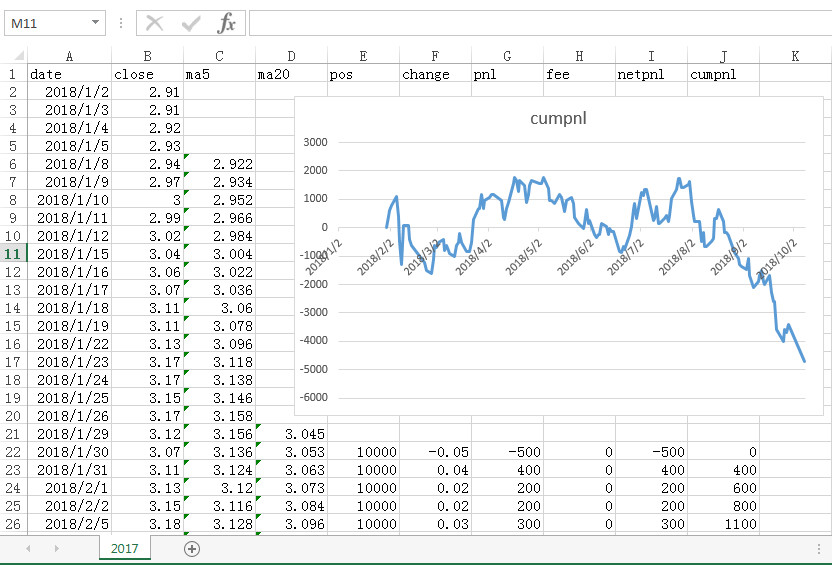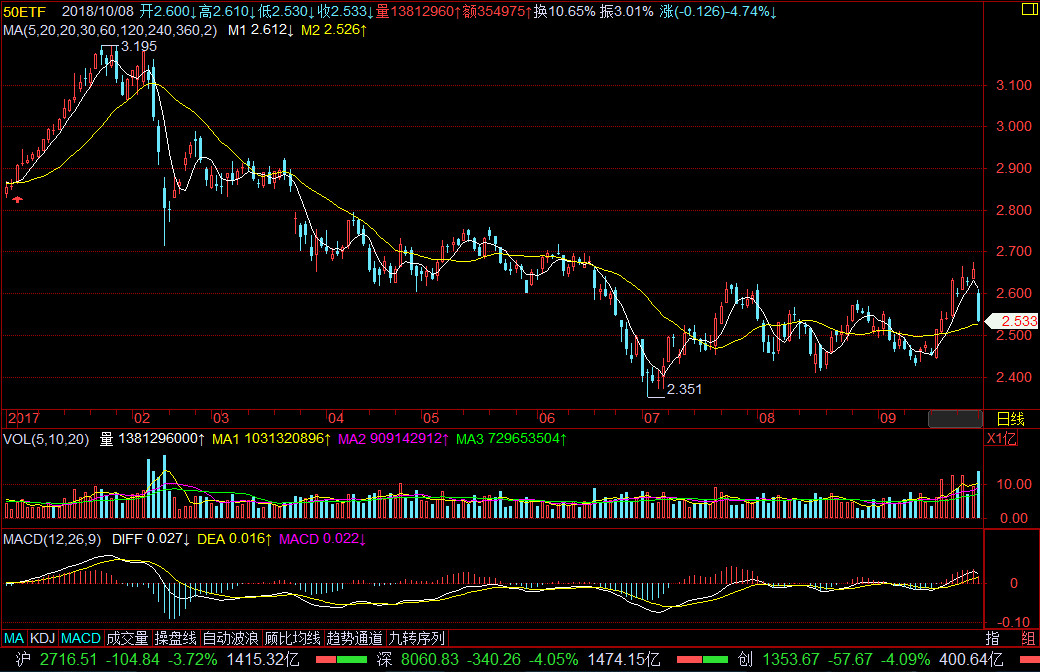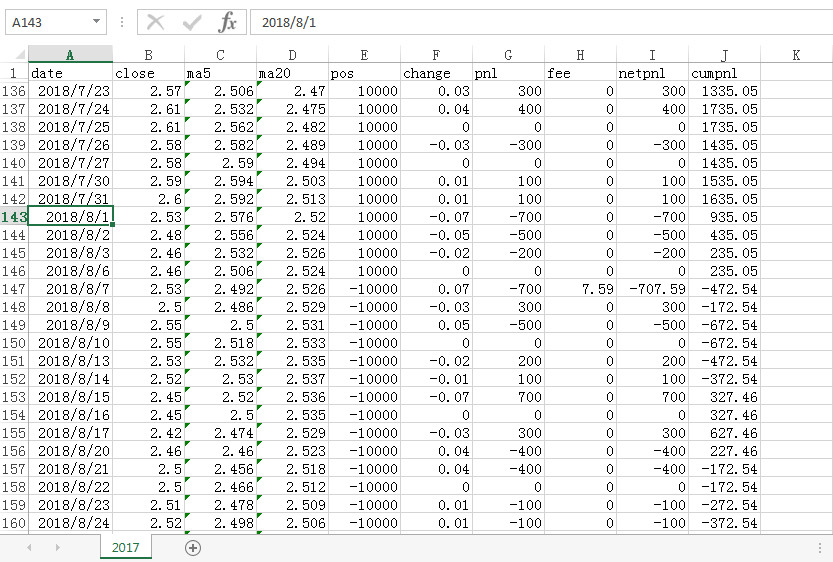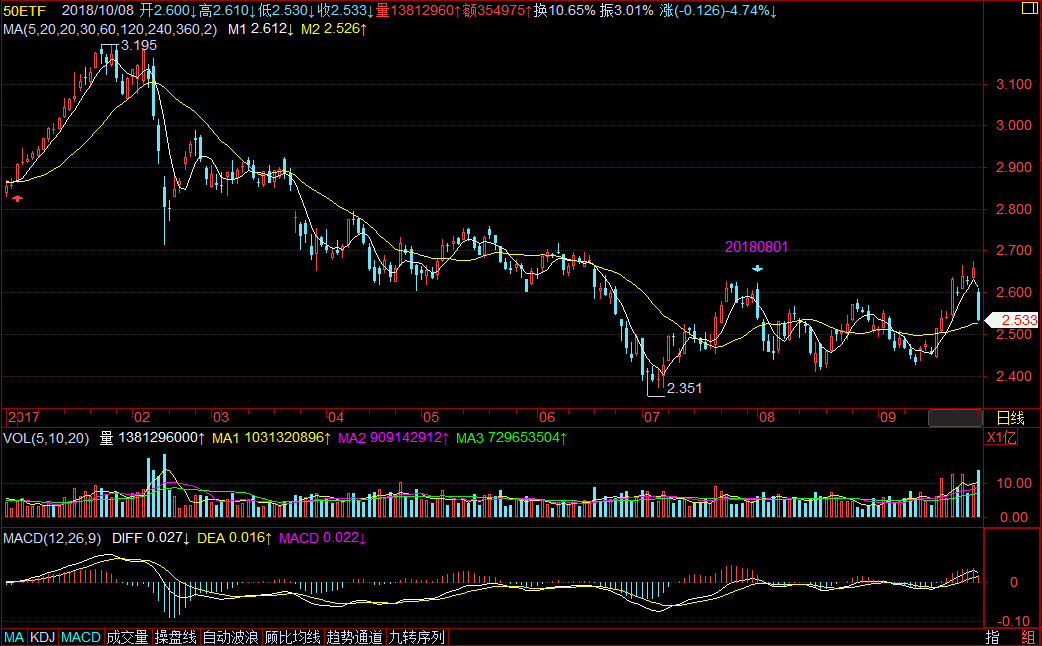1.代码

```import pandas as pd
import tushare as ts
data = ts.get_hist_data("510050",start="2017-01-01",end="2017-09-08")
data = data.sort_values("date")
df = pd.DataFrame()
df['close'] = data['close']
df['change'] = df['close']- df['close'].shift(1)
df['ma5'] = df['close'].rolling(window=5,center=False).mean()
df['ma20'] = df['close'].rolling(window=20,center=False).mean()
df = df.dropna()  #将带有Na的去掉，返回一个新的对像
df['pos'] = 0
df['pos'][df['ma5']>=df['ma20']] = 10000
df['pos'][df['ma5']<df['ma20']] = -10000
```

A value is trying to be set on a copy of a slice from a DataFrame

```df['pnl'] = df['change']*df['pos']  #盈亏
df['fee'] = 0
df['fee'][df['pos']!= df['pos'].shift(1)] = df['close']*20000*0.0003
df['netpnl'] = df['pnl'] - df['fee'] #净盈亏
df['cumpnl'] = df['netpnl'].cumsum()
df['cumpnl'].plot()
```

2、效果展示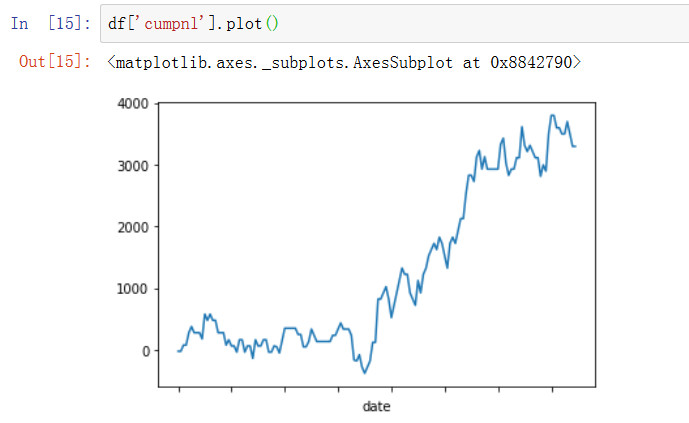```df['pos'] = df['pos'].shift(1).fillna(0)
```